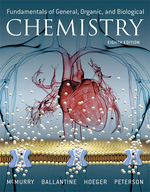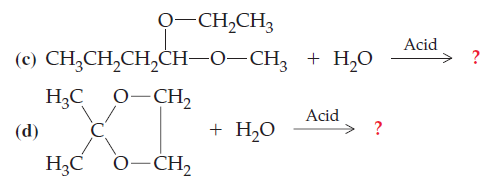×
Get Full Access to Fundamentals Of General, Organic, And Biological Chemistry (Mastering Chemistry) - 8 Edition - Chapter 15 - Problem 15.42
Get Full Access to Fundamentals Of General, Organic, And Biological Chemistry (Mastering Chemistry) - 8 Edition - Chapter 15 - Problem 15.42

×ISBN: 9780134015187 2044

## Solution for problem 15.42 Chapter 15

Fundamentals of General, Organic, and Biological Chemistry (Mastering Chemistry) | 8th Edition

• Textbook Solutions
• 2901 Step-by-step solutions solved by professors and subject experts
• Get 24/7 help from StudySoup virtual teaching assistantsFundamentals of General, Organic, and Biological Chemistry (Mastering Chemistry) | 8th Edition

4 5 1 258 Reviews
18
2
Problem 15.42

Write the structures of the hemiacetal or hemiketal that result from reactions (a) and (b). Label each product as a hemiacetal or hemiketal. Write the structures of the complete hydrolysis products of the acetal or ketal in (c) and (d).

(a) $$\text { Butan-2-one }+\text { Propan-1-ol } \longrightarrow ?$$

(b) $$\text { Butanal }+\text { Isopropanol } \longrightarrow ?$$Text Transcription:

Butan-2-one Propan-1-ol longrightarrow ?

Butanal+Isopropanol longrightarrow ?

Step-by-Step Solution:
Step 1 of 3

THERMODYNAMICS Spontaneous Processes: ● Spontaneous Process: a process that proceeds without outside intervention ● Nonspontaneous Process: process that cannot happen on its own ● Experimental conditions like temperature and pressure affect spontaneity ○ nonspontaneous process can become spontaneous under conditions like temperature and pressure ● Many exothermic reactions including combustion reactions and metal corrosion are spontaneous ○ some exothermic reactions aren’t spontaneous and some endothermic reactions are spontaneous ○ depends on reaction conditions ● Endothermic reactions are spontaneous due to their particles being more spread out and/or having more freedom of motion than the particles in their reactants Entropy: ● When an isolated thermodynamic system undergoes a change where the particles in the system disperse into a larger volume and/or gain freedom of motion, the process is always spontaneous ○ System experiences an increase in entropy ● Second law of thermodynamics: entropy of an isolated thermodynamic system always increases during a spontaneous process ● Entropy (S): a measure of how dispersed the energy of a system is ● Energy Dispersion ○ In the lab experiment, energy initially confined to the metal flows spontaneously from the hot metal into the cool water and is dispersed throughout the metal/water system

Step 2 of 3

Step 3 of 3

##### ISBN: 9780134015187

The answer to “?Write the structures of the hemiacetal or hemiketal that result from reactions (a) and (b). Label each product as a hemiacetal or hemiketal. Write the structures of the complete hydrolysis products of the acetal or ketal in (c) and (d).(a) $$\text { Butan-2-one }+\text { Propan-1-ol } \longrightarrow ?$$(b) $$\text { Butanal }+\text { Isopropanol } \longrightarrow ?$$ Text Transcription:Butan-2-one Propan-1-ol longrightarrow ?Butanal+Isopropanol longrightarrow ?” is broken down into a number of easy to follow steps, and 65 words. This full solution covers the following key subjects: . This expansive textbook survival guide covers 281 chapters, and 240 solutions. This textbook survival guide was created for the textbook: Fundamentals of General, Organic, and Biological Chemistry (Mastering Chemistry), edition: 8. The full step-by-step solution to problem: 15.42 from chapter: 15 was answered by Aimee Notetaker, our top Chemistry solution expert on 04/25/22, 03:57PM. Since the solution to 15.42 from 15 chapter was answered, more than 204 students have viewed the full step-by-step answer. Fundamentals of General, Organic, and Biological Chemistry (Mastering Chemistry) was written by Aimee Notetaker and is associated to the ISBN: 9780134015187.

## Discover and learn what students are asking

Calculus: Early Transcendental Functions : Basic Differentiation Rules and Rates of Change
?How Do You See It? Use the graph of f to answer each question. To print an enlarged copy of the graph, go to MathGraphs.com. (a) Betwee

Statistics: Informed Decisions Using Data : Discrete Random Variables
?What is a random variable?

Statistics: Informed Decisions Using Data : Testing the Significance of the Least-Squares Regression Model
?Why don’t we conduct inference on the linear correlation coefficient?

Statistics: Informed Decisions Using Data : Inference on the Least-Squares Regression Model and Multiple Regression
?State the requirements to perform inference on a simple least-squares regression line

Unlock Textbook Solution